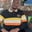Related Tags

swift
communitycreator

# What are operators in Swift?Wale Moshood

Operators are special symbols used to perform various operations on variables and values. Swift provides a range of operators found in already known languages such as C and improves on their functionalities.

Operators in Swift are divided into several categories based on the kinds of operations they perform.

### Arithmetic operators

The four standard arithmetic operators are supported for all number types in Swift. Aside from the four standard arithmetic operators, they also give support to the Modulo or Remainder operator.

The Modulo operator is an arithmetic operator that returns the remainder of an integer division. The Modulo operator is denoted with the $%$ symbol and can only be used with integers.

The 5 arithmetic operators in Swift are:

• Subtraction
• Multiplication
• Division
• Modulo

These operators are described in the example below:

let x = 11
let y = 3

print (x + y)

// subtraction
print (x - y)

// integer division
print (x / y)

// multiplication
print (x * y)

// modulo
print (x % y)  

The division operator only returns the quotient if the two numbers to be divided are integers. The division operator in the example above exhibits this behavior. However, if the division operator is used to divide floats, meaning floating-point numbers, the exact result will be returned.

An example is shown below:

let x = 7.0
let y = 2.0

// float division
print (x / y)  

### Assignment operators

Assignment operators are used to initialize or update the values of variables. Assignment operators are denoted with the = symbol.

The syntax below assigns the value of $10$ to the variable a.

var a = 10

Assignment operators can be of various types, which include:

• Subtraction assignment
• Division assignment
• Multiplication assignment
• Modulo assignment

These operators are used in the example below:

// Assigns 7 to a
var a = 7

// Assigns 2 to b
var b = 2

// Addition Assignment - assigns the sum of a and b to a
a += b      // a = a + b

// Subtractiontion Assignment - assigns the difference between a and b to a
a -= b      // a = a - b

// Division Assignment - assigns the quotient after the division between a and b to a
a /= b      // a = a / b

// Multiplication Assignment - assign the product of a and b to a
a *= b      // a = a * b

// Modulo Assignment - assign the remainder after the division between a and b to a
a %= b      // a = a % b

### Comparison operator

Comparison operators are used to compare two variables or values. Comparison operators return a Boolean depending on the result and are used in decision-making and loops. Swift supports different comparison operators, such as the following:

• Greater than (>)
• Less than (<)
• Greater than or equal to (>=)
• Less than or equal to (<=)
• Equal to (==)
• Not equal to (!=)

The example below demonstrates how to use these comparison operators:

var a = 9

var b = 2

// Greater than operator
print(a > b)

// Less than operator
print(a < b)

// Greater than or equal to operator
print(a >= b)

// Less than or equal to operator
print(a <= b)

// Equal to operator
print(a == b)

// Not equal to operator
print(a != b)

### Logical operators

Logical operators are important in decision-making. They are used to combine the Boolean logic values and check if an expression is true or false.

The logical operators supported by Swift are:

• Logical AND - denoted with (&&) returns true only if both operands are true. Else, it returns false.
• Logical OR - denoted with (||) returns true if at least one of the operands is true. Else, it returns false.
• Logical NOT - denoted with (!) returns true if the operand is false and vice-versa.

An example is shown below:

let firstCondition = true

let secondCondition = false

print(firstCondition && secondCondition)

print(firstCondition || secondCondition)

print(!secondCondition) 

### Other operators in Swift

• Range operators - Used in loops to define a range of values.
• Bitwise operators - Used to carry out operations on individual bits.
• Ternary operators - Return value based on specific conditions.

RELATED TAGS

swift
communitycreator

CONTRIBUTORWale Moshood
RELATED COURSES

View all Courses

Keep Exploring

Learn in-demand tech skills in half the time